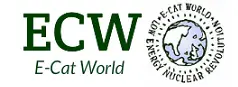# Determining the Fuel Composition for an E-Cat Reactor (Steven Karels)

The following post was submitted by Steven Karels

Based on the Lugano Report, an estimate of the fuel composition may be attempted.

Known facts:

1. Fuel sample had a mass of 1 gram
2. Page 29: “From the analysis methods of the fuel we find that there are significant quantities of Li, Al, Fe and ICP-AES analysis we find there is about 0.011 grams of 7Li in the 1 gram fuel.”
3. Page 29: “… the information from ICP-AES that there is about 0.55 gram NI in the fuel.”
4. Page 28: “From all combined analysis methods of the fuel we find that there are significant quantities of Li, Al, Fe and H in addition to the Ni.”
5. Page 28: “… from the ICP-AES analysis which shows the mass ratio between Li and Al is compatible with a LiAlH4 molecule.”
6. Page 28: “…natural composition, i.e. 6Li 7% and 7Li 93%”
7. Page 28: “We remark in particular that hydrogen but no deuterium was seen by SIMS.”

Analysis

The average mass of the lithium atoms are 0.07*6 + 0.93*7 = 6.93 amu.
Aluminum atoms have a mass of 27 amu while hydrogen atoms have an average mass of 1.
So the molecular weight of the LiAlH4 must be 6.93 + 27 + 4 = 37.93 amu.
There for the amount of LiAlH4 must be 0.011 grams * 37.93 / 6.93 = 0.06 grams and the amount of aluminum must be 0.043 grams. The amount of hydrogen in the LiAlH4 must be 0.006 grams.
The iron mass must therefore be 1.0 grams (total) – 0.55 grams (Ni) – 0.043 grams (Al) – 0.011 grams (Li) – 0.006 grams (H) = 0.39 grams of iron.

Element % by Weight
Nickel 55.0
Iron 39.0
Aluminum 4.3
Lithium 1.1
Hydrogen (no Deuterium) 0.6
Total 100.0
LiAlH4 6.0

It is also likely that the LiAlH4 was prepared using hydrogen depleted of deuterium.